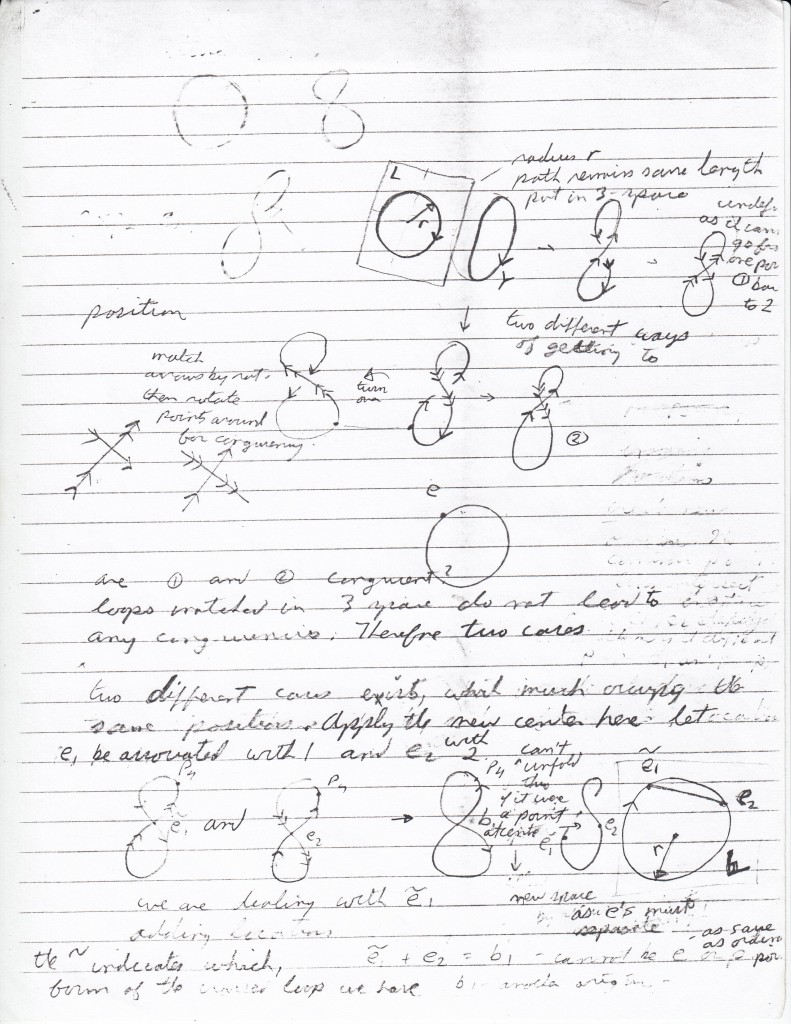# Interactive Online Tutoring Services

## April 29, 2016

### Second page of notes

This second note starts with a picture of a circle with radius r with a direction in the space modelled after the overlapping shadows.

The indicated locations can move through the three-dimensional space of placements and locations to eventually create a self-intersecting loop of locations. In order to do this a continuous series of joinings are gone through and a circle of voids, or just placements is left.

But we can also have the usual motion of a loop through space to give a self-intersecting loop. We can call this a move of the diagram, while in the other case we can call this a shift of the diagram. So the extra background gives us two different ways of altering the diagram.

What we can do then is move the diagram to create a self-intersecting loop in the usual sense. But I can reach this self-intersecting loop in two possible ways either by twisting through space one way or the other.

What I can do is create one diagram which covers both cases. If I place the center, as created in the first note at the self-intersection point of the self-intersecting  loop and then let the loop unloop itself but this time by shifting, not by moving. Then the multiple point b(1) has components e(1) and e(2) and e(1) and e(2) can switch positions. Then this models both twist cases.